# 方法概述与定义

## 方法介绍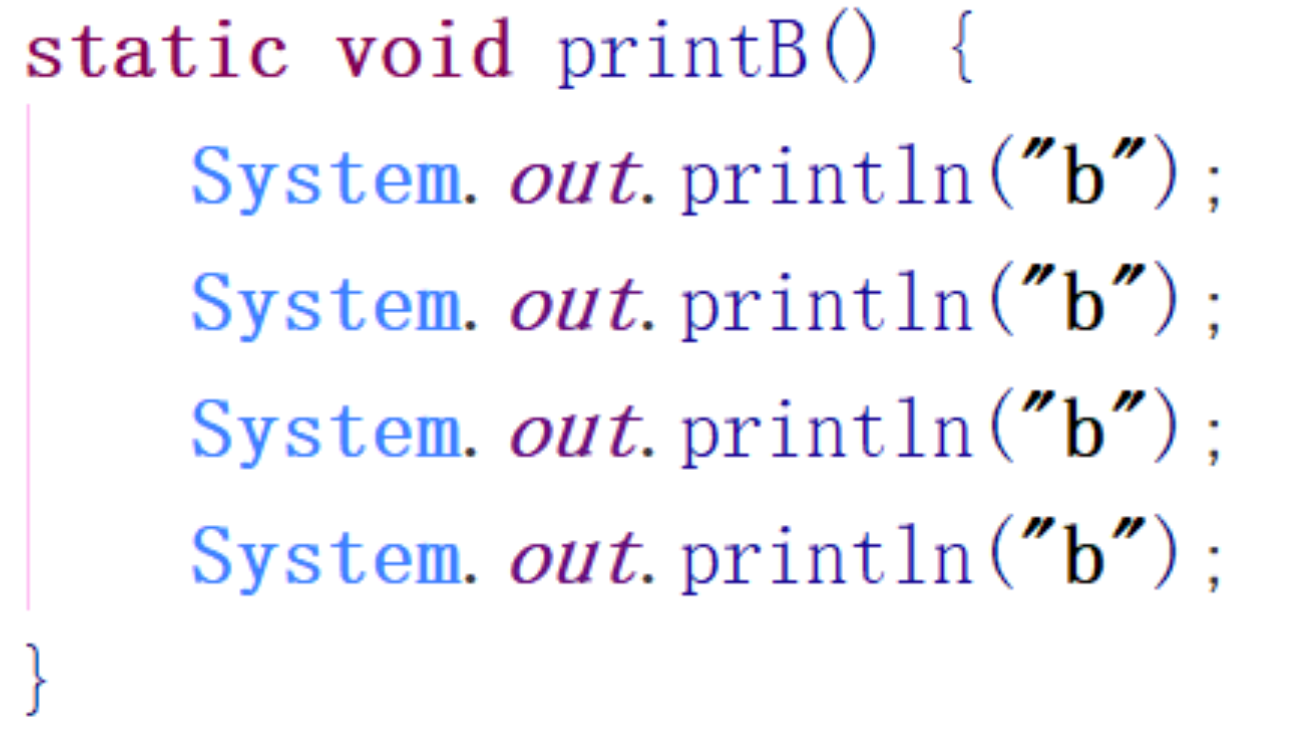static void printB() {
System.out.println("b");
System.out.println("b");
System.out.println("b");
System.out.println("b");
}


## 方法注意点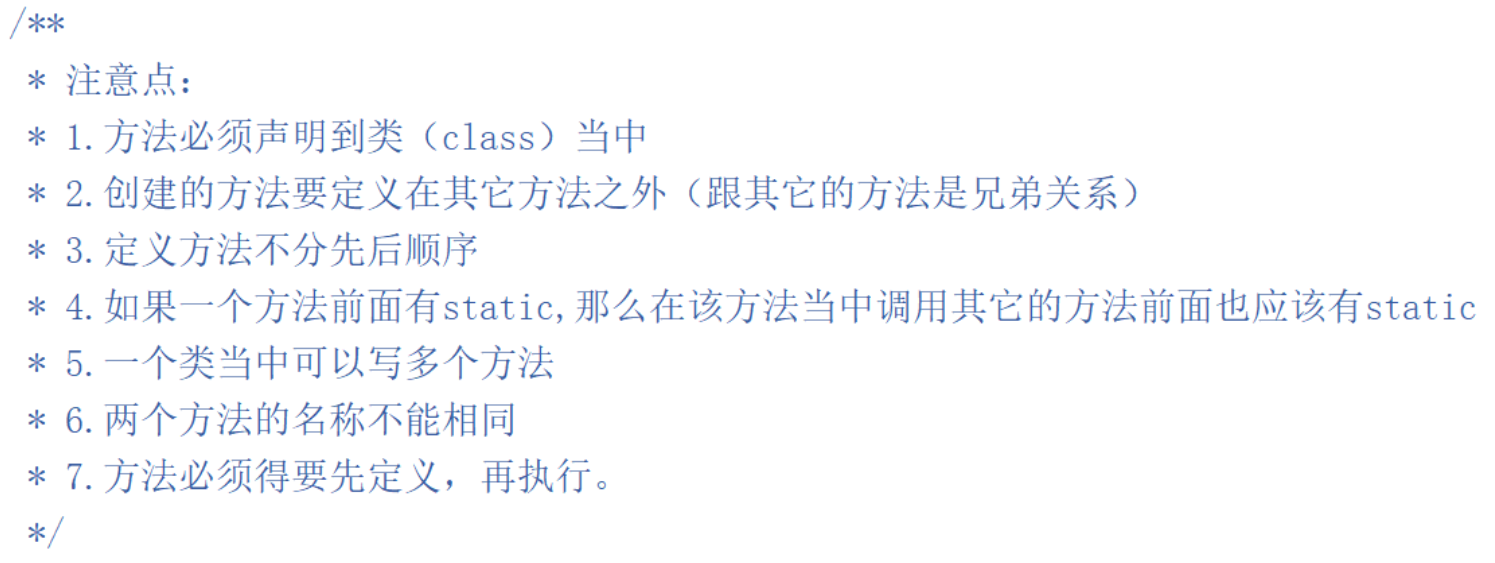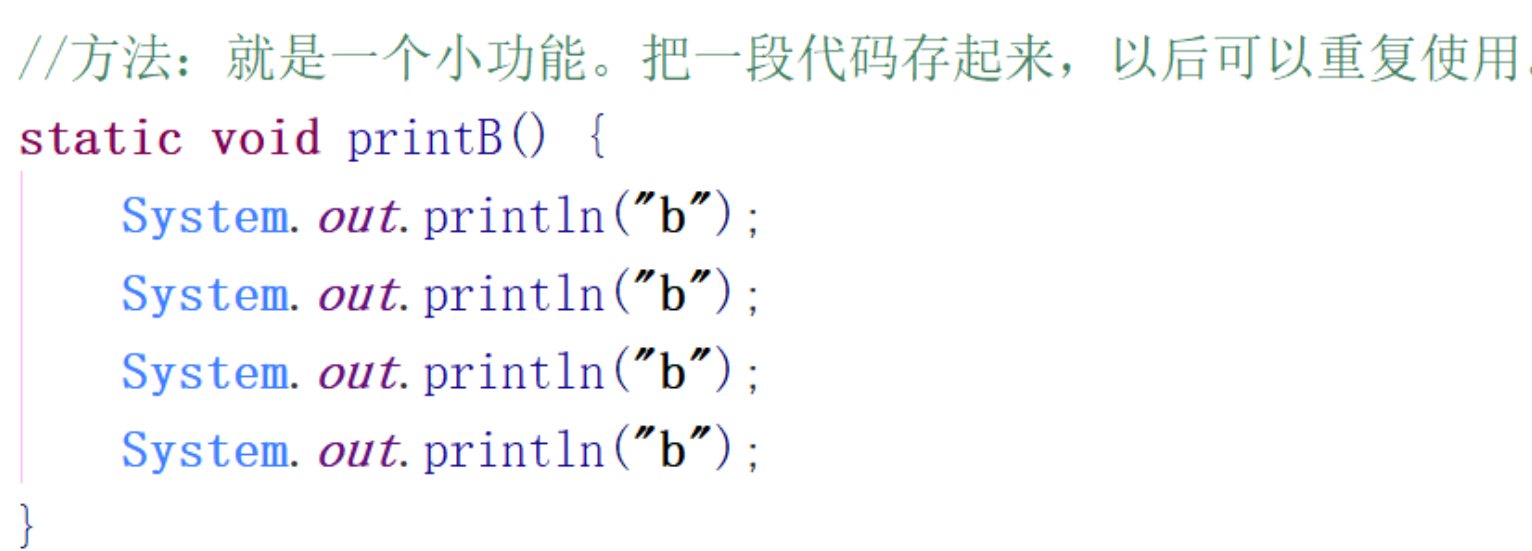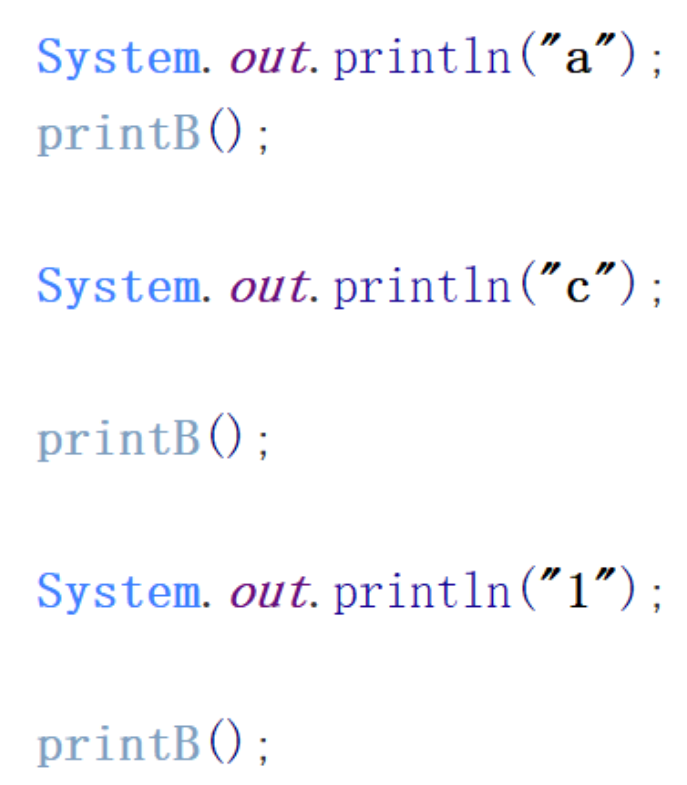## 方法参数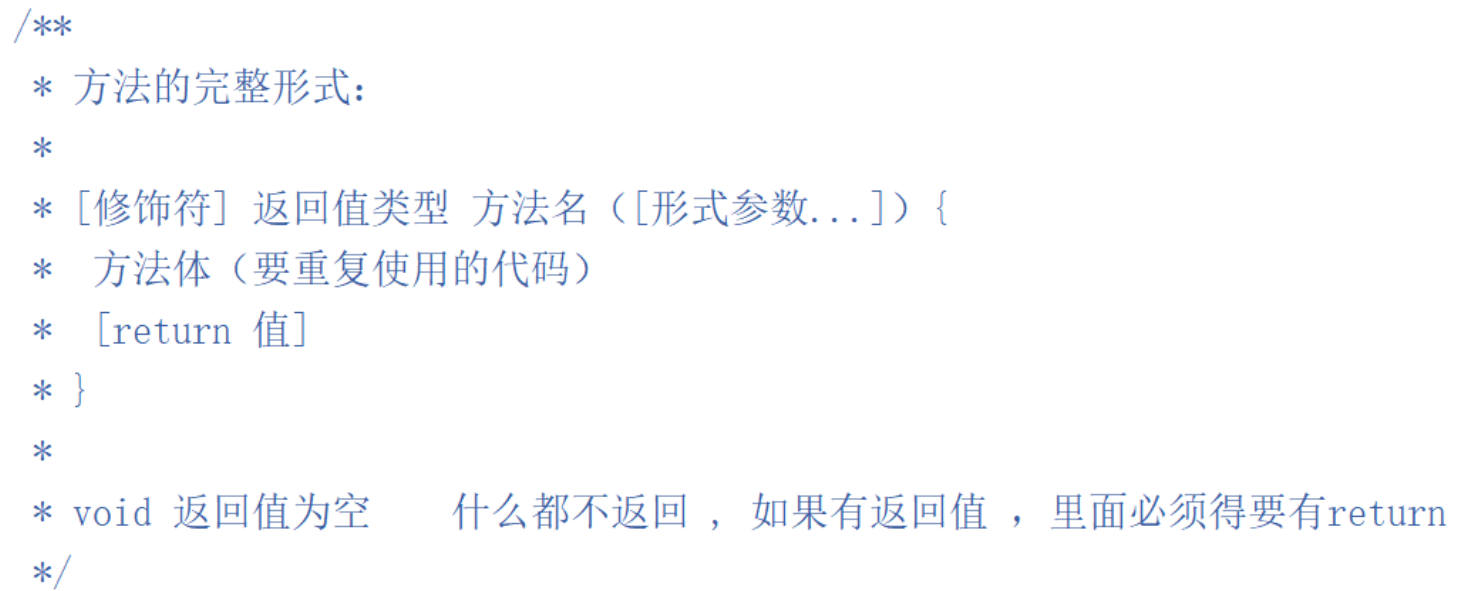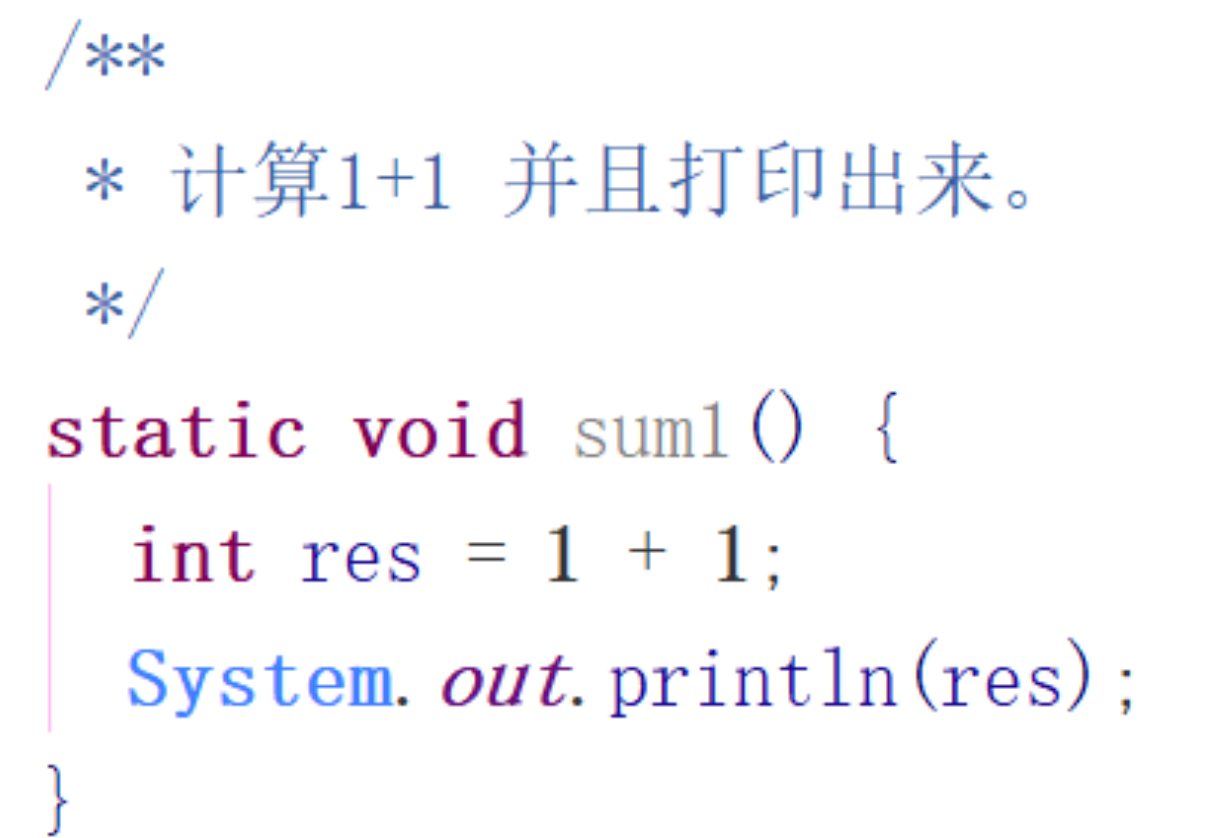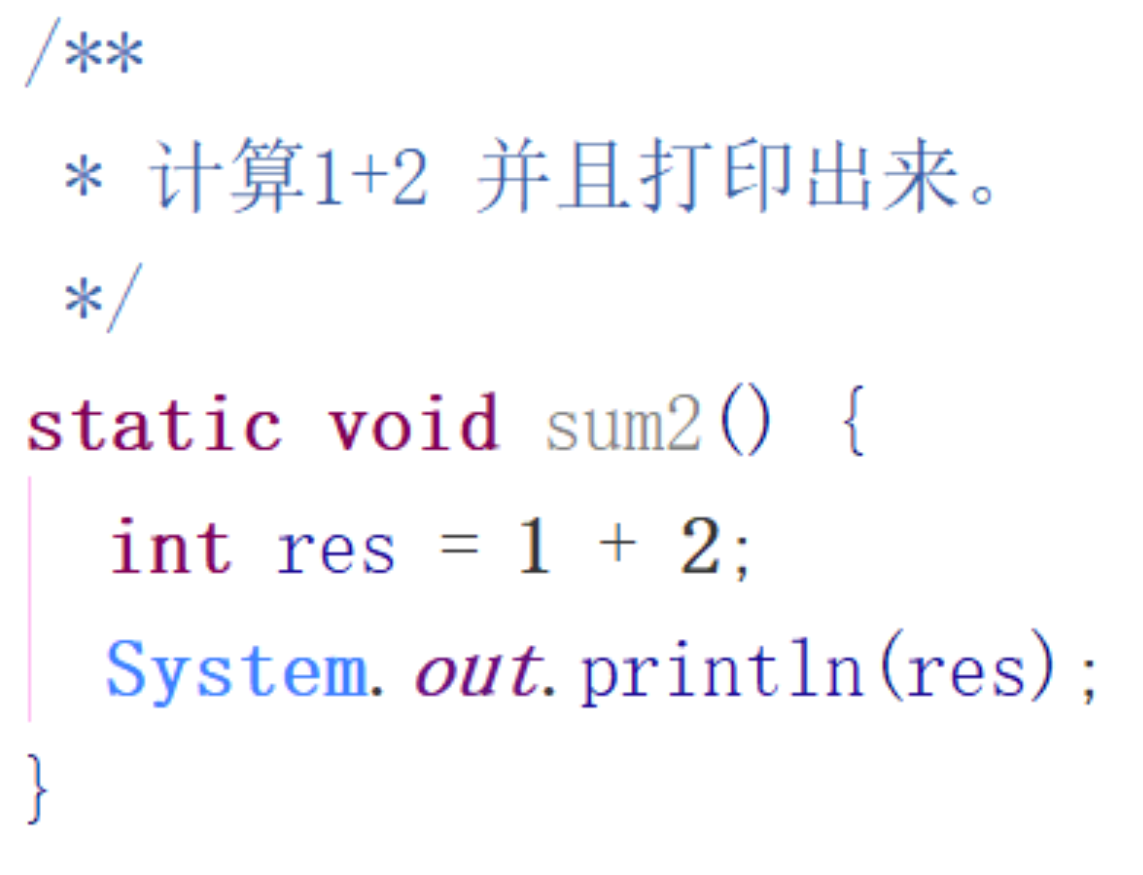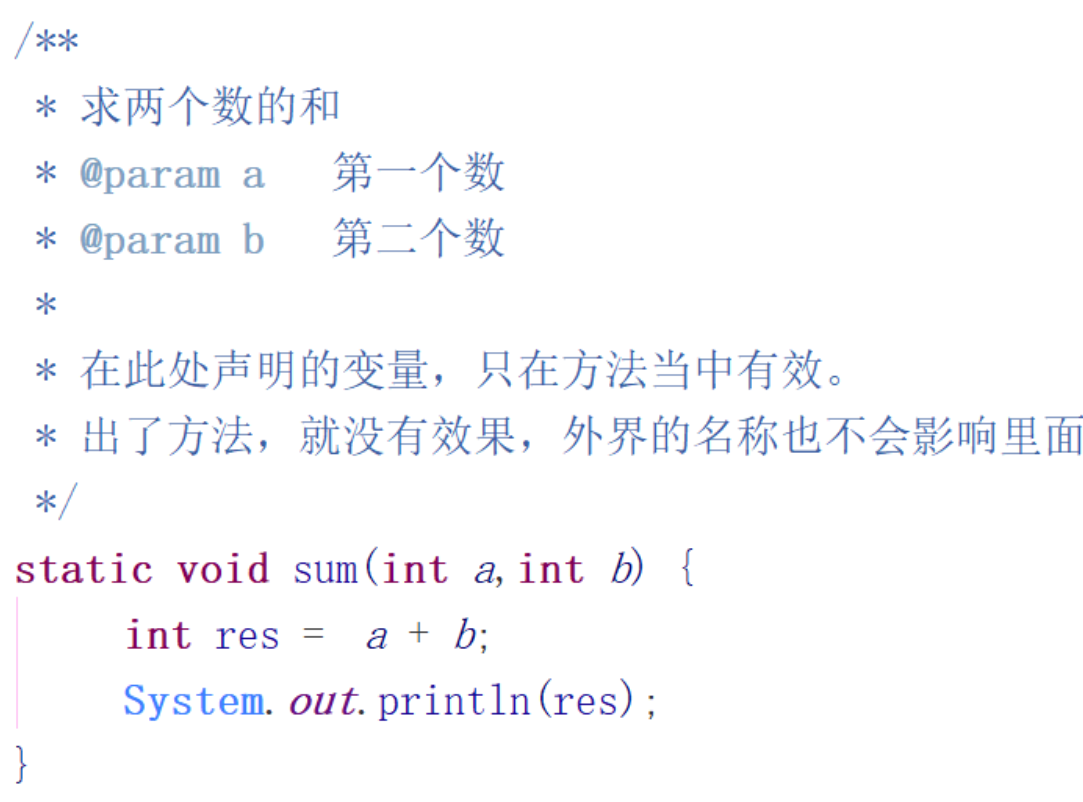## 方法返回值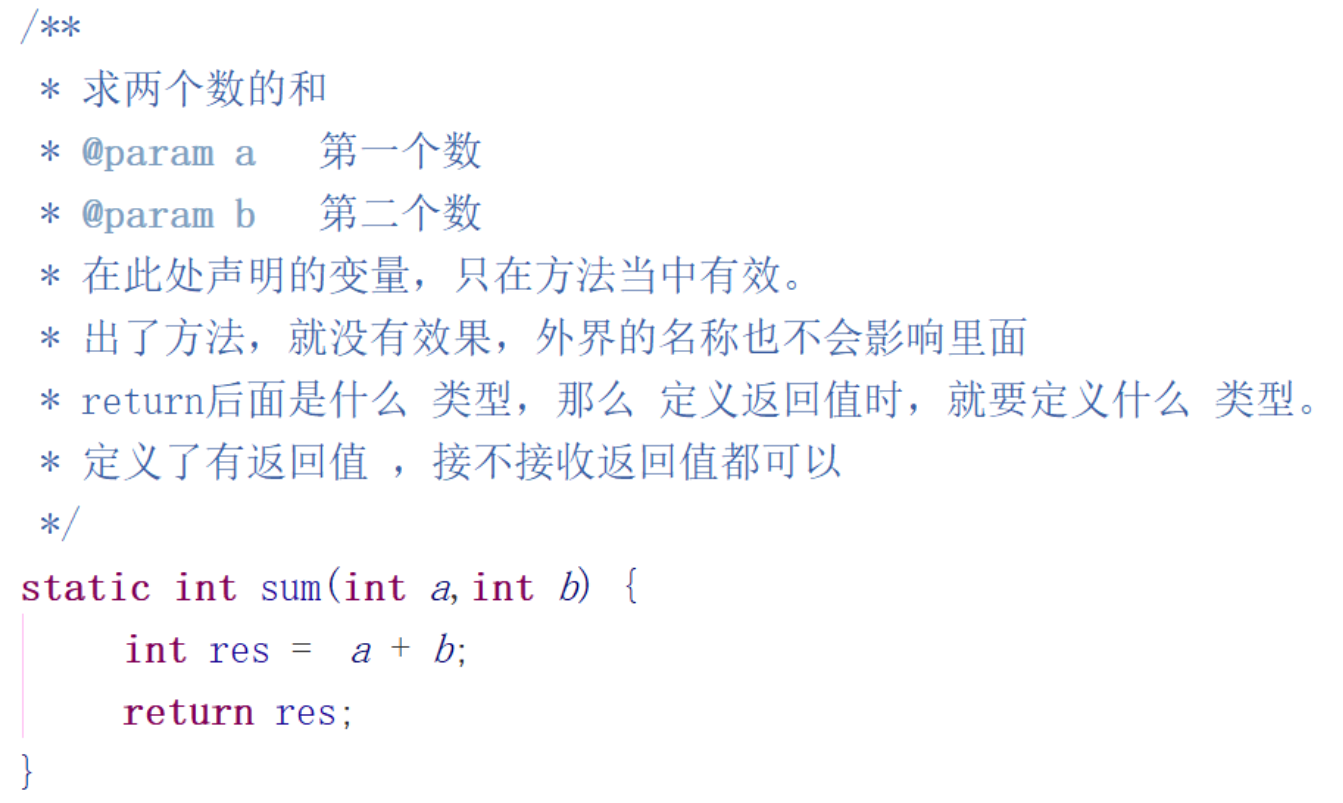## 驼峰命名法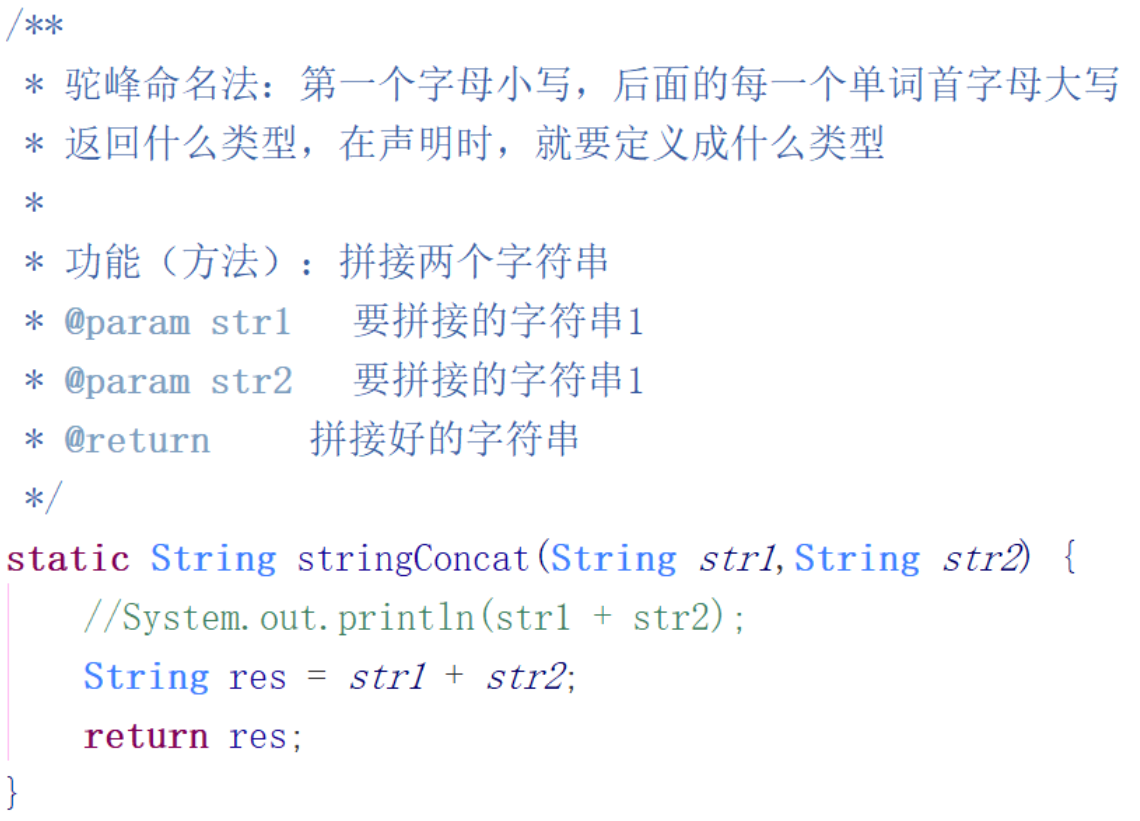static String stringConcat(String str1,String str2) {
//System.out.println(str1 + str2);
String res = str1 + str2;
return res;
}


# 方法重载

## 方法的重载的规则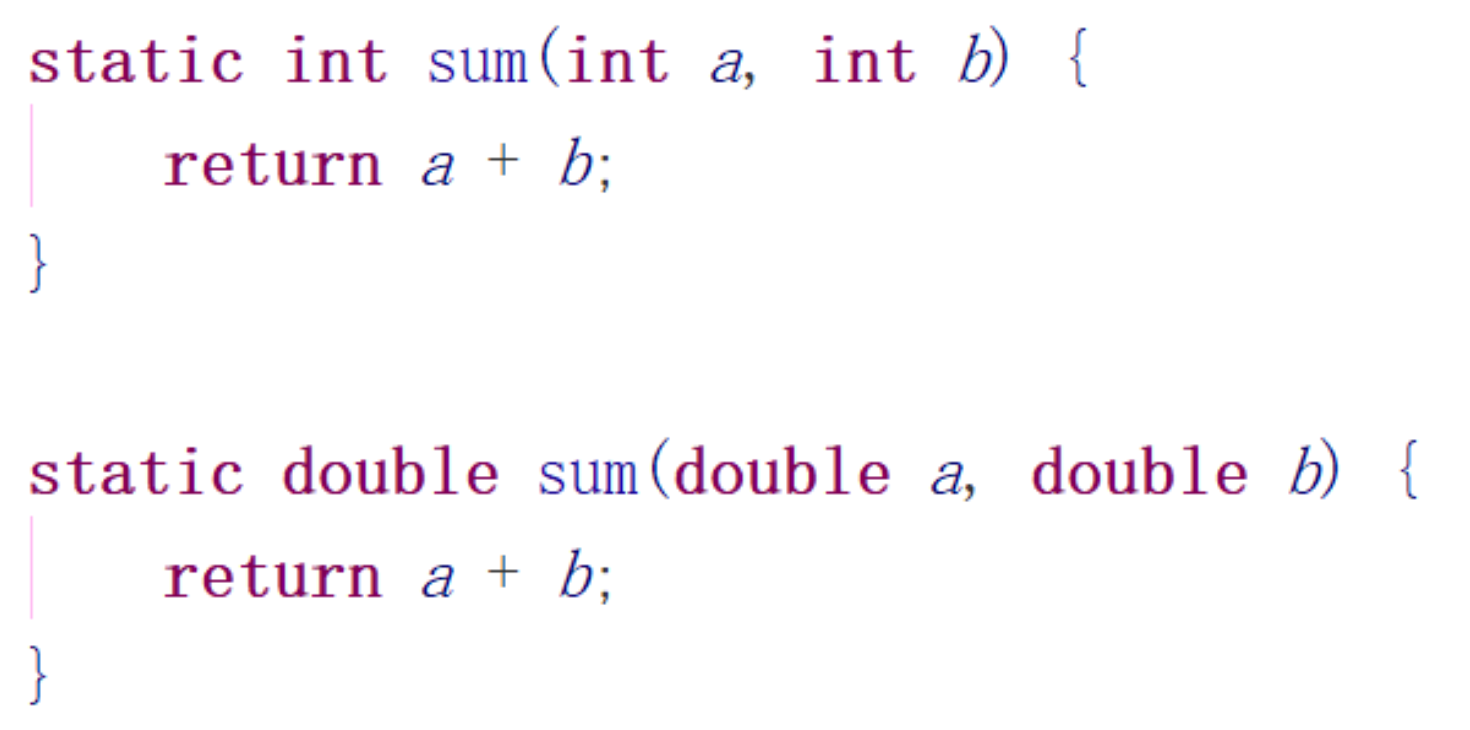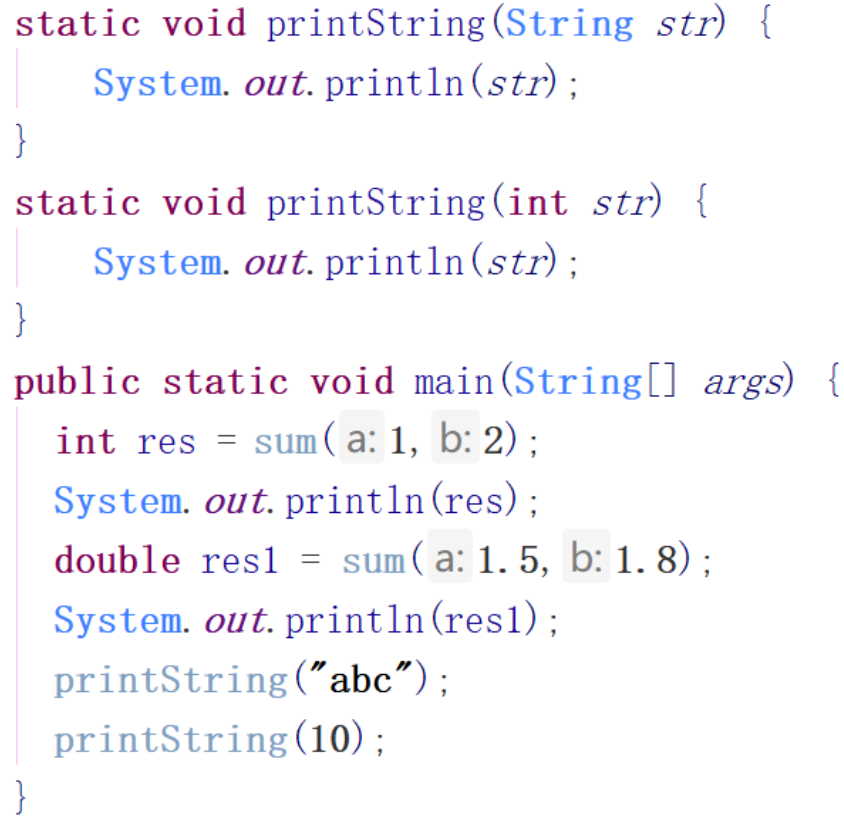# 数组

## 格式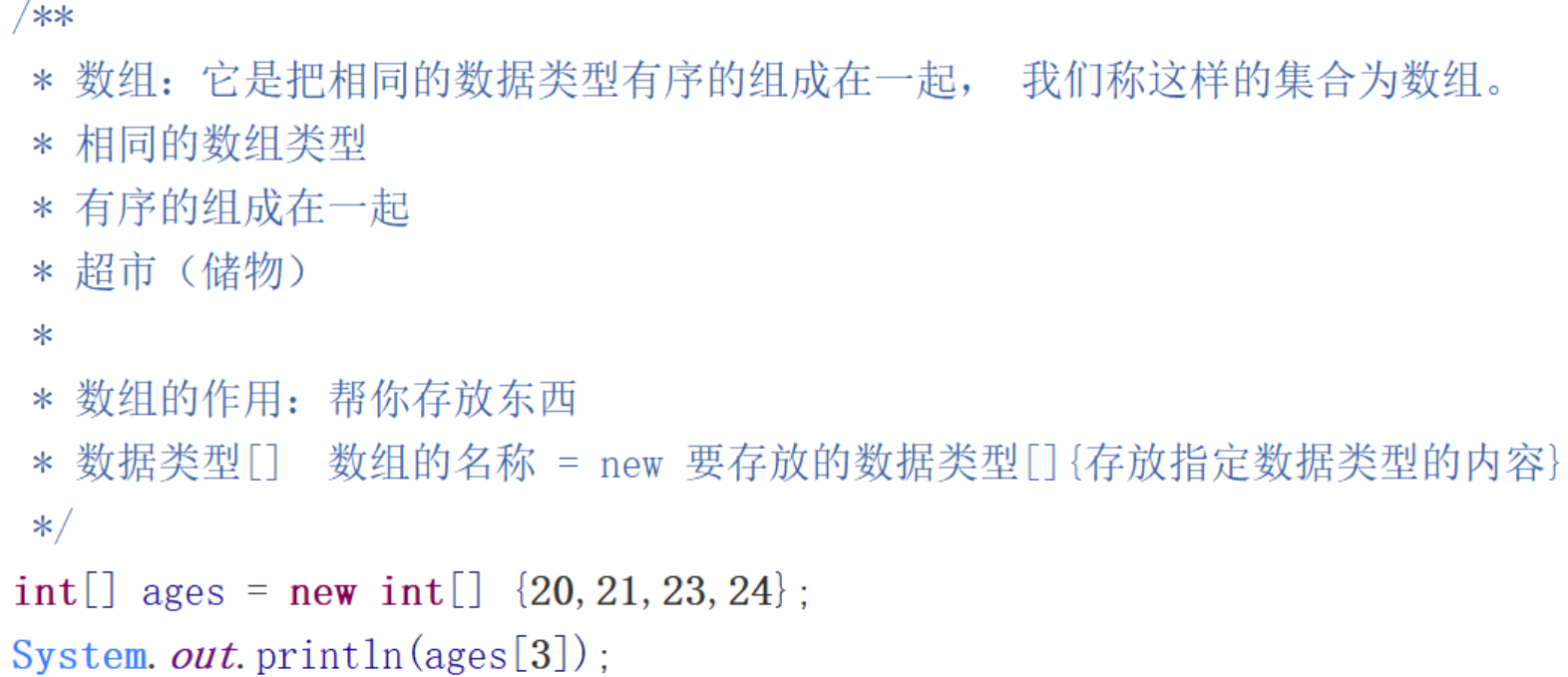## 规则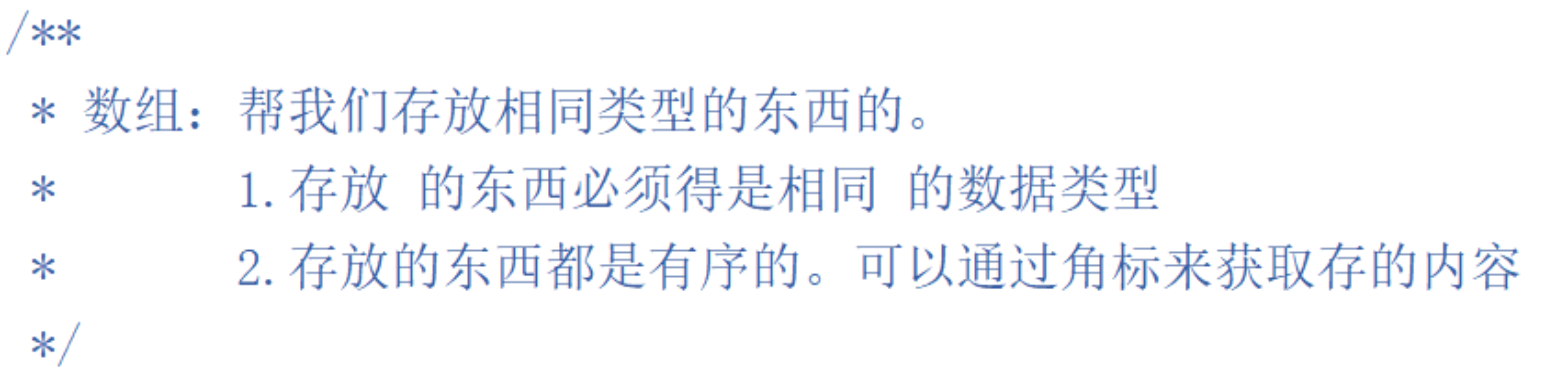## 数组初始化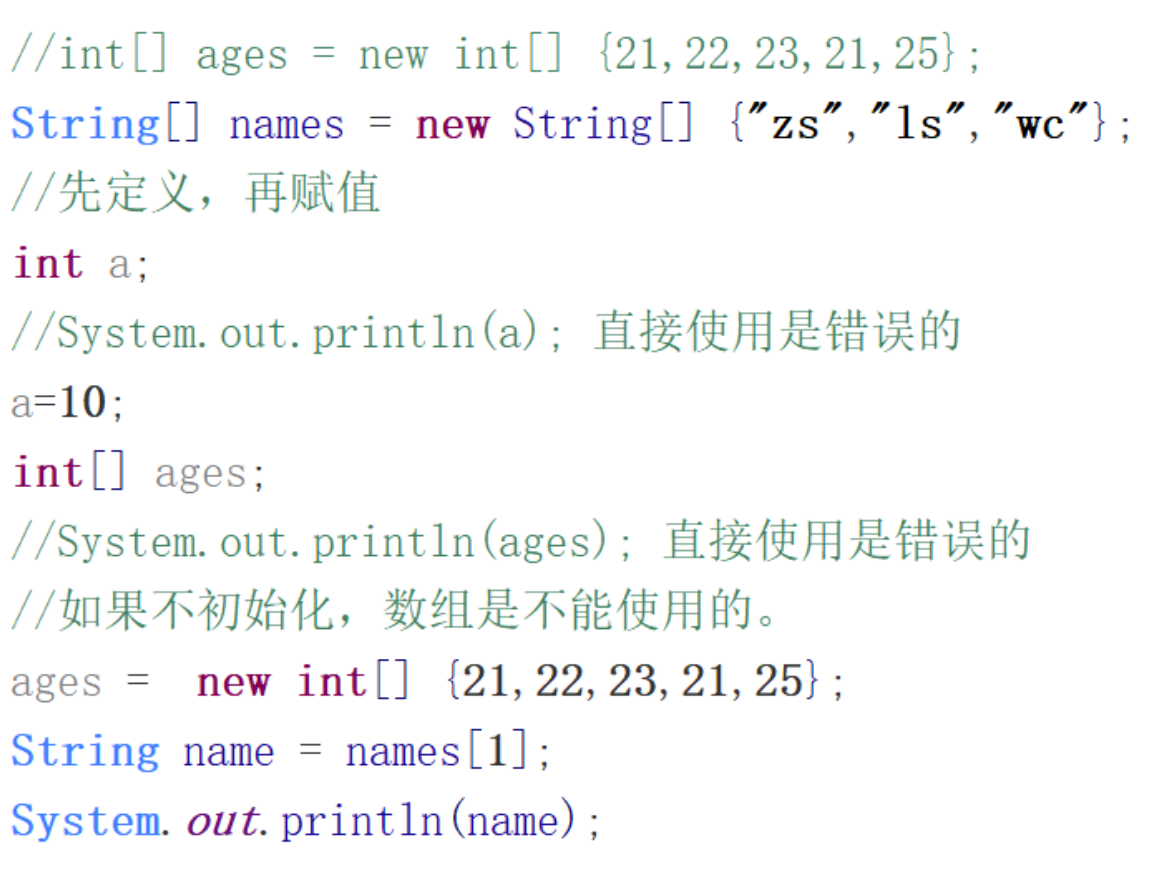//int[] ages = new int[] {21,22,23,21,25};
String[] names = new String[] {"zs","ls","wc"};
//先定义，再赋值
int a;
//System.out.println(a); 直接使用是错误的
a=10;
int[] ages;
//System.out.println(ages); 直接使用是错误的
//如果不初始化，数组是不能使用的。
ages =  new int[] {21,22,23,21,25};
String name = names;
System.out.println(name);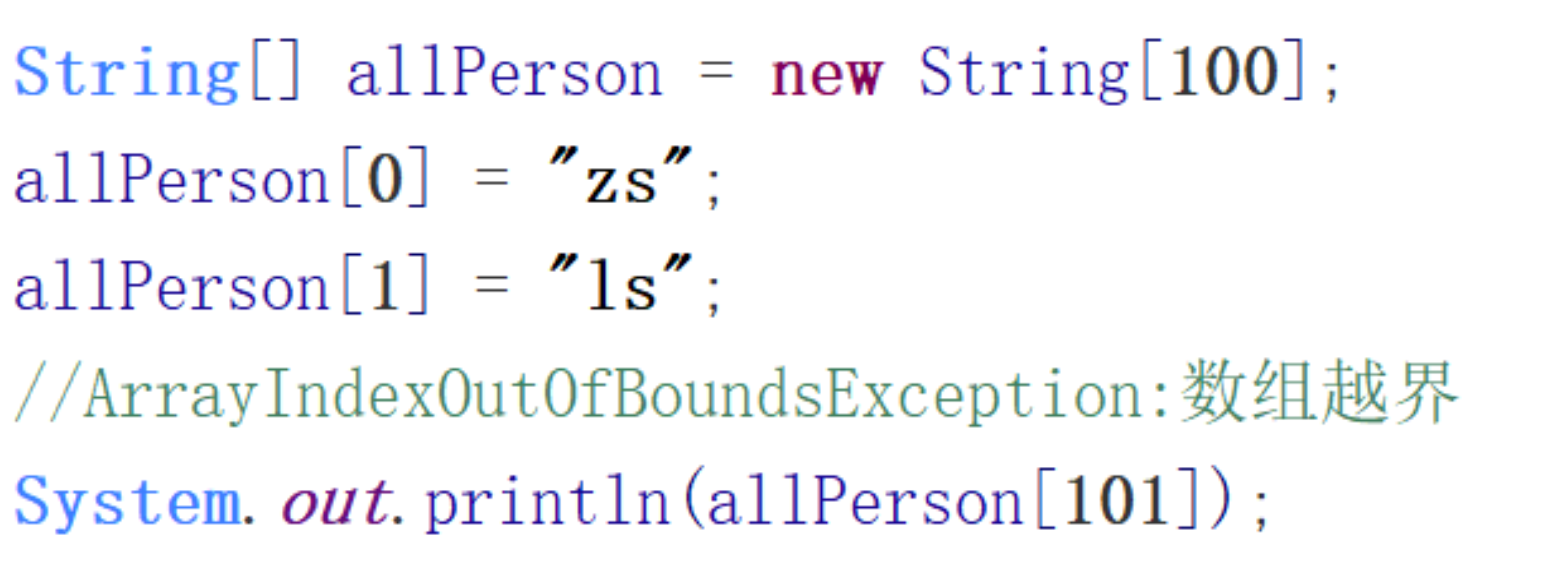String[] allPerson = new String;
allPerson = "zs";
allPerson = "ls";
//ArrayIndexOutOfBoundsException:数组越界
System.out.println(allPerson);


## 数组长度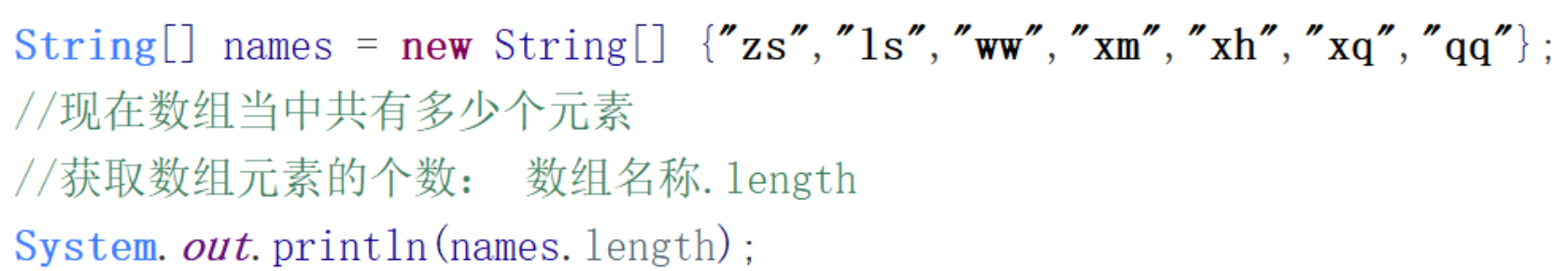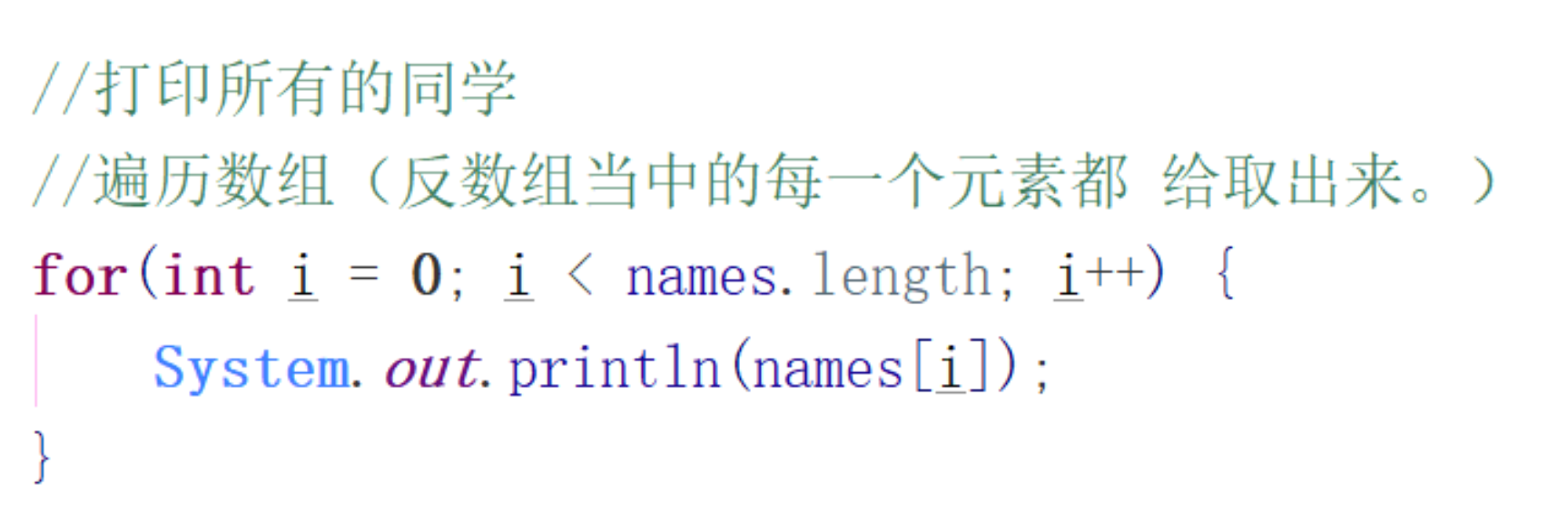## 排序算法

### 冒泡排序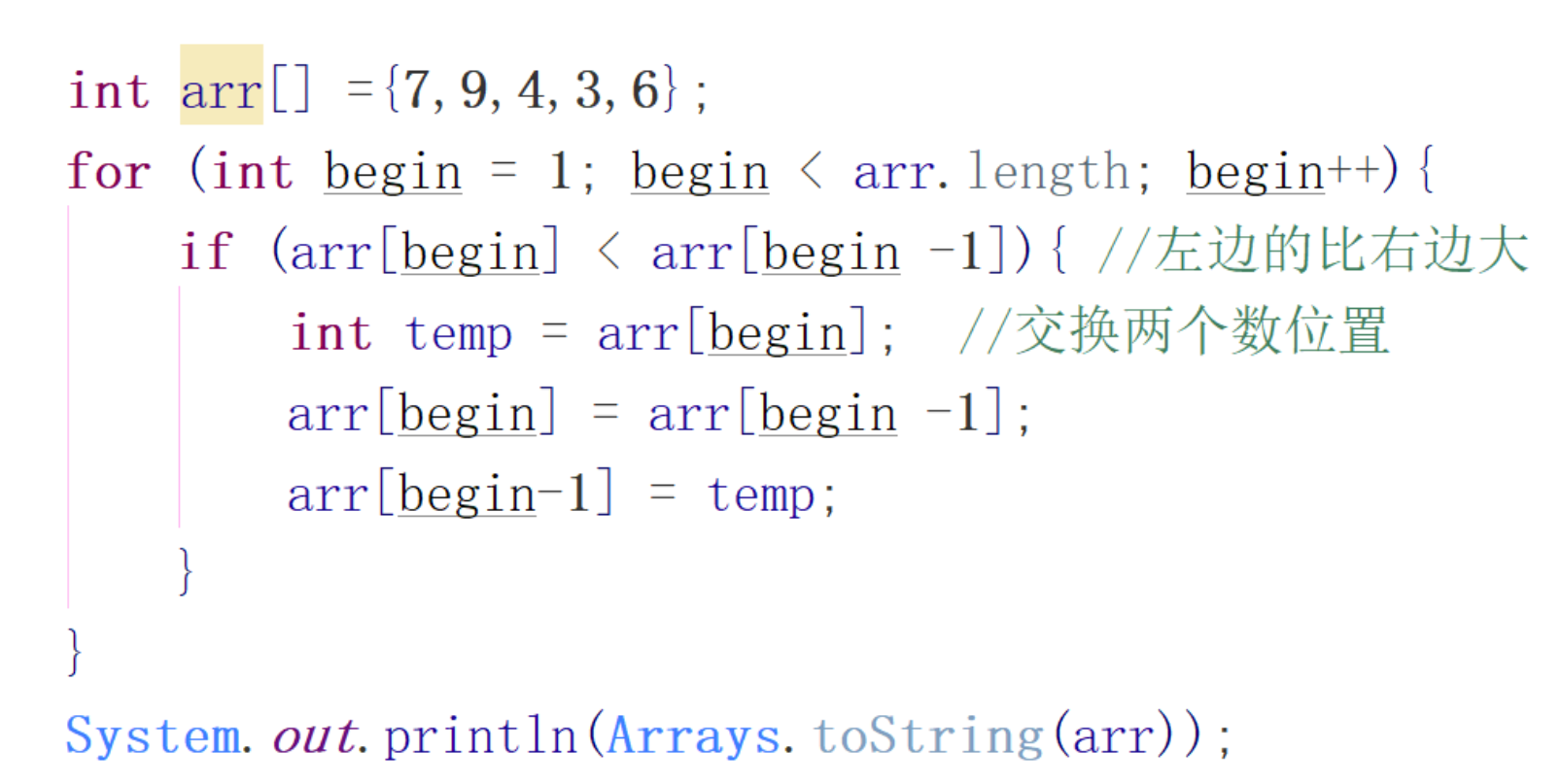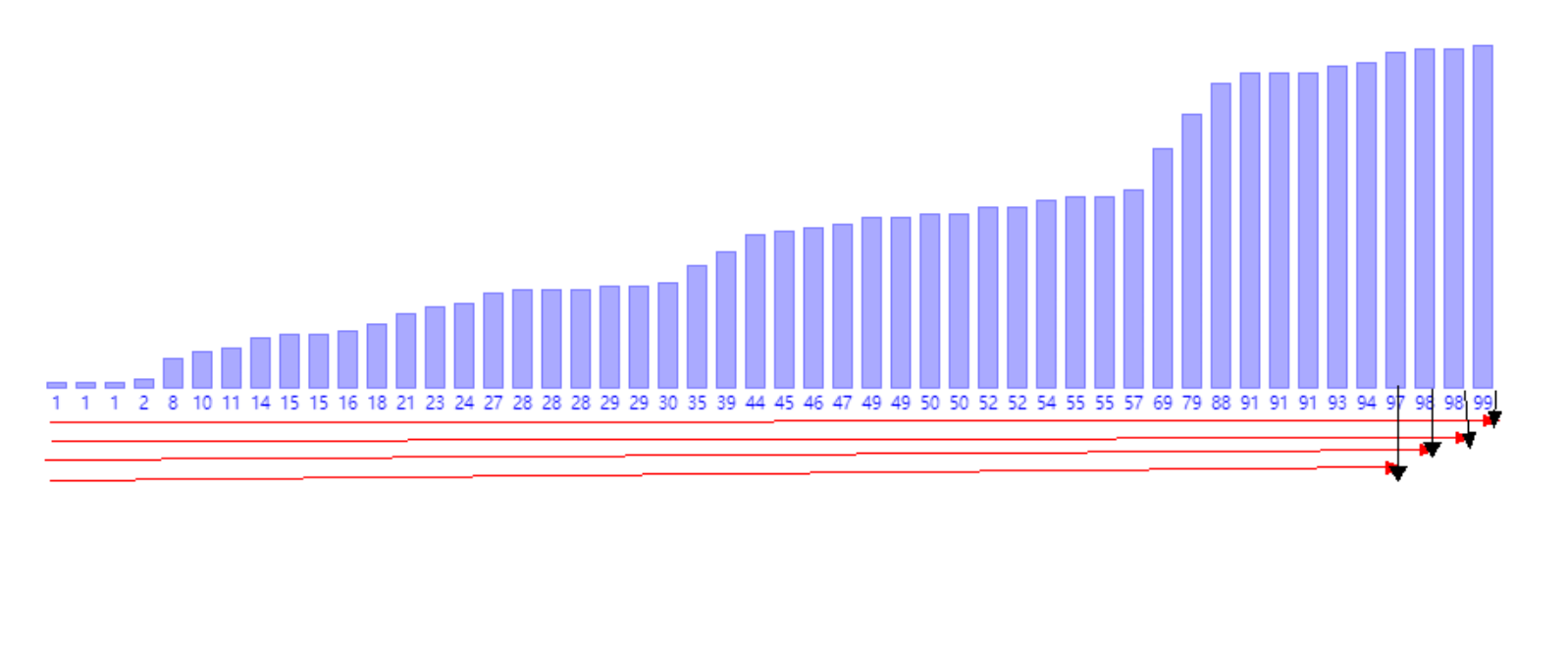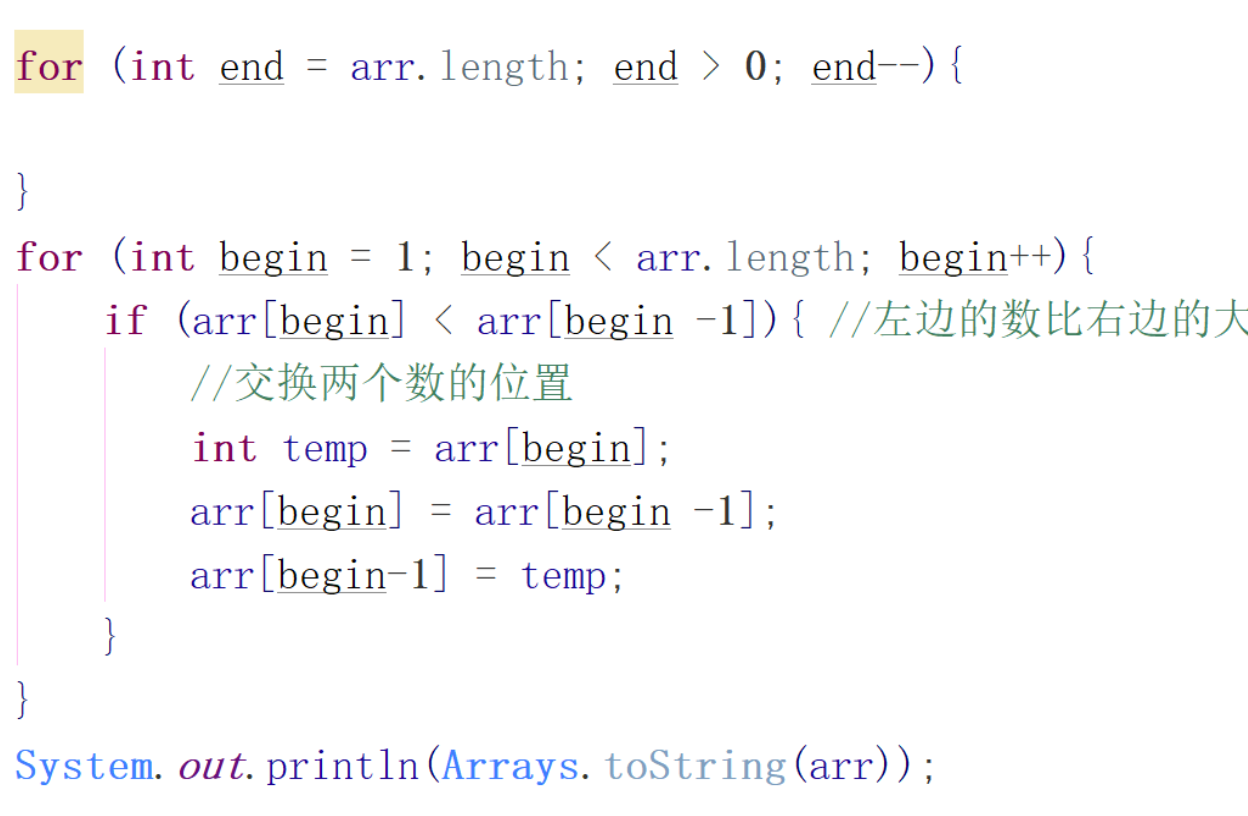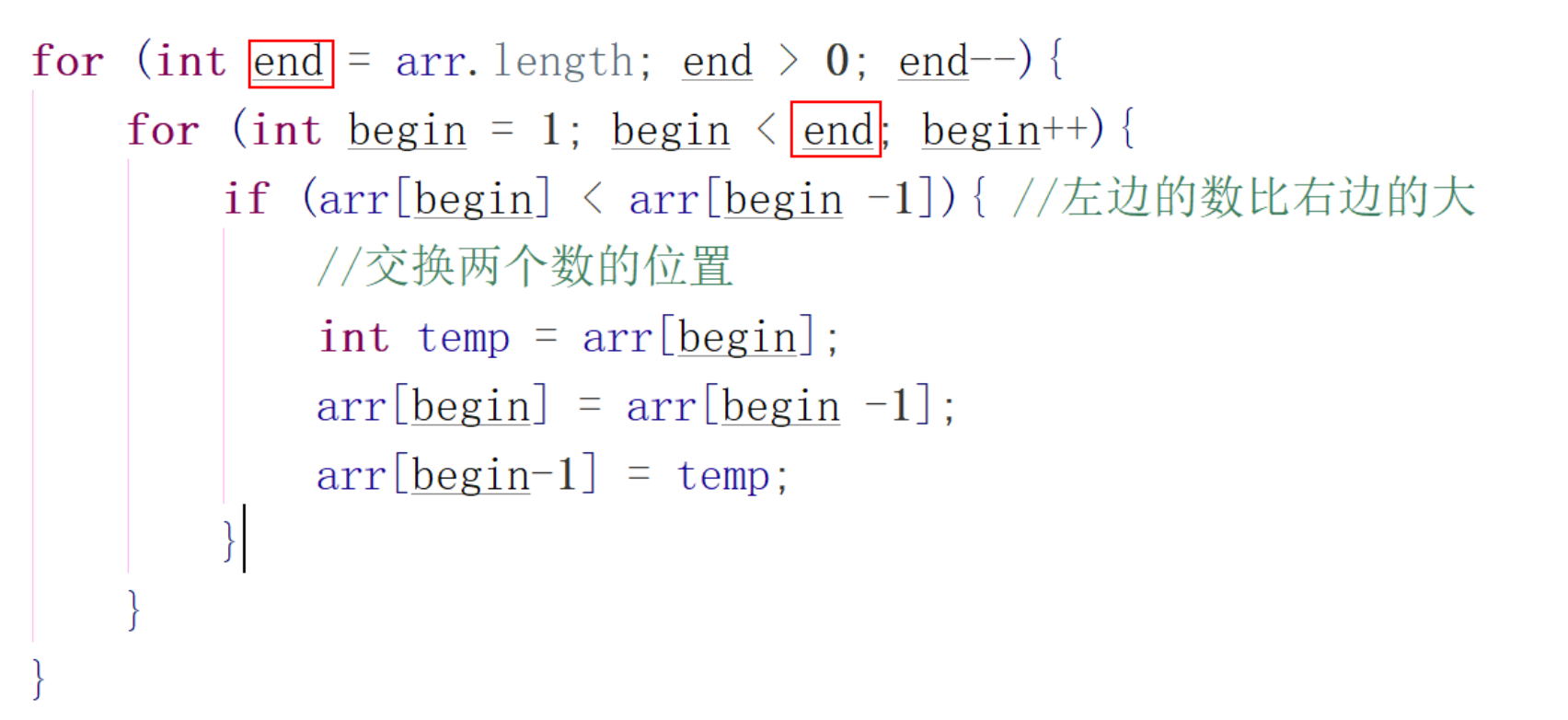int arr[] ={7,4,3,6,9};
for (int end = arr.length; end > 0; end--){
for (int begin = 1; begin < end; begin++){
if (arr[begin] < arr[begin -1]){ //左边的数比右边的大
//交换两个数的位置
int temp = arr[begin];
arr[begin] = arr[begin -1];
arr[begin-1] = temp;
}
}
}
System.out.println(Arrays.toString(arr));


### 选择排序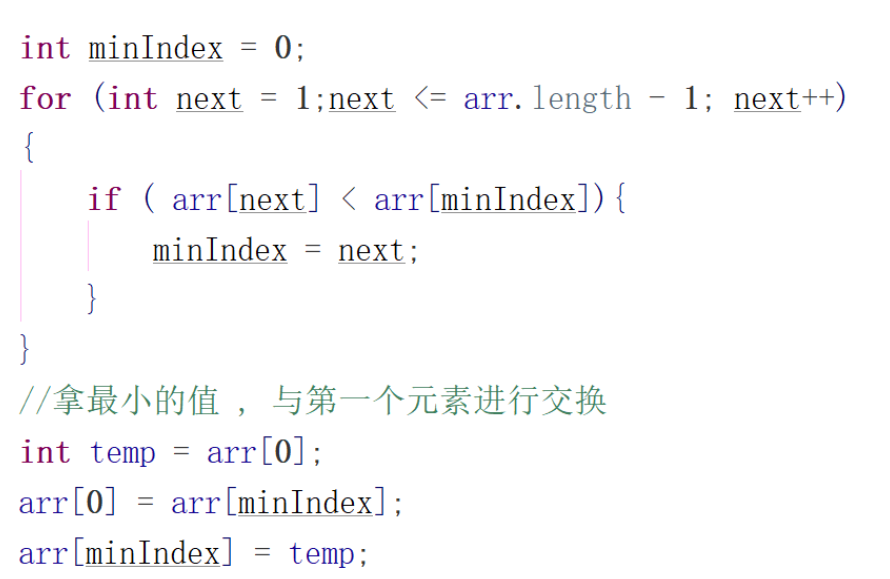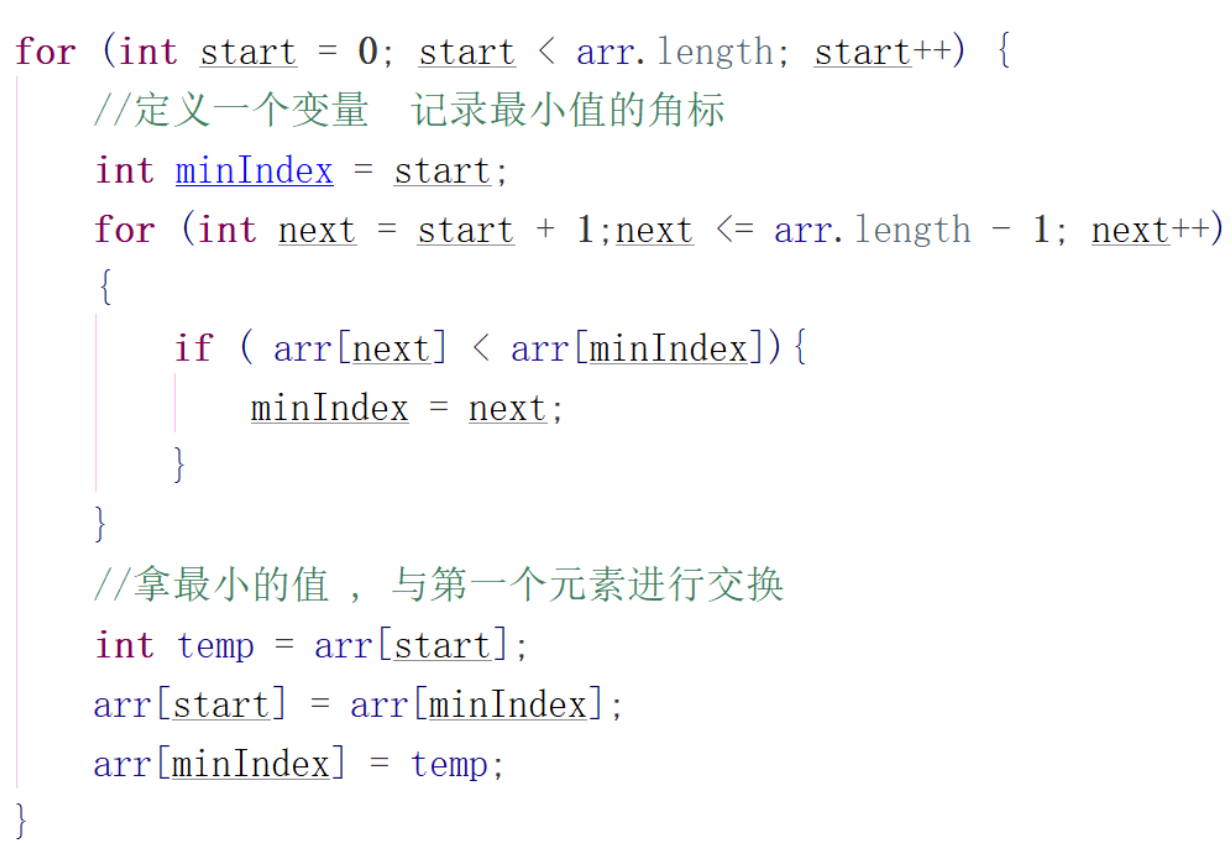for (int start = 0; start < arr.length; start++) {
//定义一个变量  记录最小值的角标
int minIndex = start;
for (int next = start + 1;next <= arr.length - 1; next++)
{
if ( arr[next] < arr[minIndex]){
minIndex = next;
}
}
//拿最小的值 , 与第一个元素进行交换
int temp = arr[start];
arr[start] = arr[minIndex];
arr[minIndex] = temp;
}


# 二分查找算法

• 必须为顺序存储结构
• 必须按关键字大小有序排列

## 原理

### 原理分析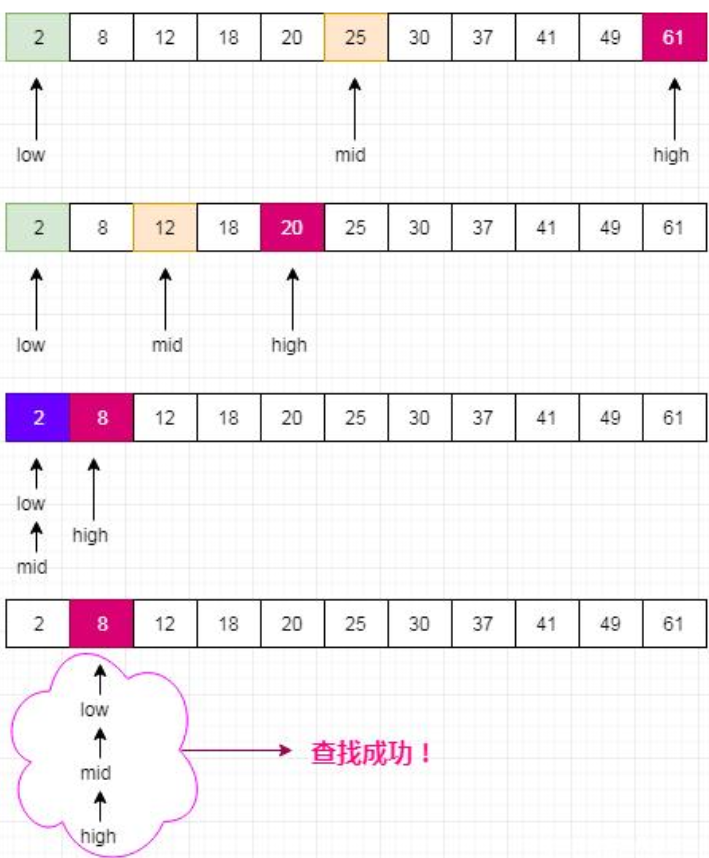## 代码实现

 public static int binarySearch(int[] nums, int target) {
int low = 0;
int high = nums.length - 1;
if (low > high || target > nums[high] || target < nums[low]){
return -1;
}
while(low <= high) {
//取中间值
int mid = (high + low) / 2;
//等于则退出查找
if(nums[mid] == target)
return mid;
else if (nums[mid] < target)
//小于则在中间值前进行比较
low = mid + 1;
else if (nums[mid] > target)
//大于在在中间值后进行比较
high = mid - 1;
}
return -1;
}


# 二维数组

## 格式

int[] classAge1 =  {18,19,20,17};
int[] classAge2 =  {18,19,20};
int[] classAge3 =  {18,19};
//整个年级的年龄
//int[][] allClassAges = new int[][] {classAge1,classAge2,classAge3};
int[][] allClassAges =  new int[][] {
{18,19,20,17},
{19,19,20},
{30,19}
};


## 二维数组遍历

for(int i = 0; i <  allClassAges.length; i++) {
int[] array = allClassAges[i];
for(int j = 0; j < array.length; j++) {
System.out.println(array[j]);
}
}


# 快速遍历

## 普通遍历

int[] ages = {10,20,30};
//遍历数组
for(int i = 0; i < ages.length; i++) {
System.out.println(i);
System.out.println(ages[i]);
}


## 快速遍历

//遍历数组foreach
//for (数组类型  遍历出来的元素 : 数组的名称)
for (int i : ages) {  //是一个语法糖
System.out.println(i);
}
String[] names = {"zs","ls","xq","123"};
for (String name : names) {
System.out.println(name);
}


# 可变参数

## 数组当作参数传递

static void sum(int[]args) {  //把数组当作参数进行传递
int res = 0;
for(int i  = 0; i < args.length; i++) {
res = res + args[i];
}
System.out.println(res);
}


## 可变参数

static void sum(int...args) {  //语法糖
int res = 0;
for(int i  = 0; i < args.length; i++) {
res = res + args[i];
}
System.out.println(res);
}

public static void main(String[] args) {
int[] params = {1,2,3,4};
sum(params);
sum(1,2,3,4);
}


# 变量作用域

## 变量作用域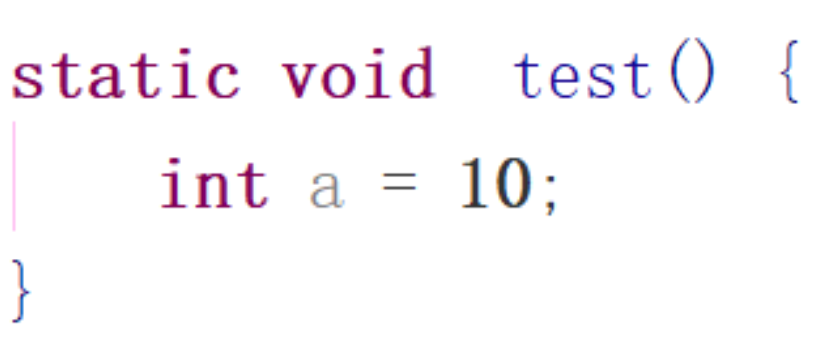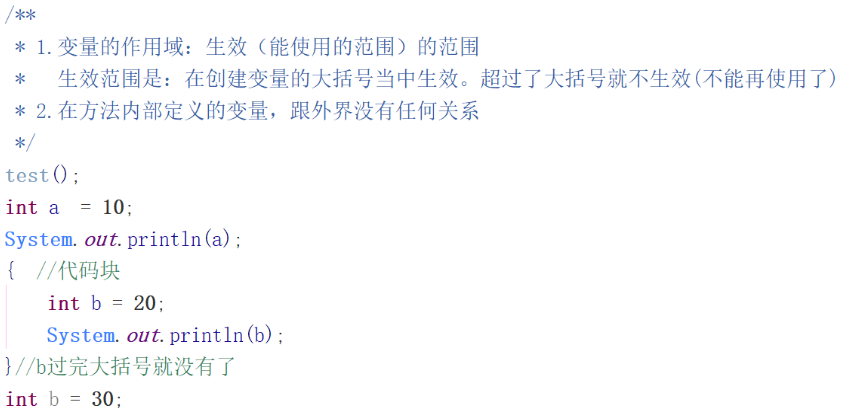test();
int a  = 10;
System.out.println(a);
{  //代码块
int b = 20;
System.out.println(b);
}//b过完大括号就没有了
int b = 30;

posted @ 2020-05-23 16:42  Leader_TBlog  阅读(78)  评论(0编辑  收藏  举报